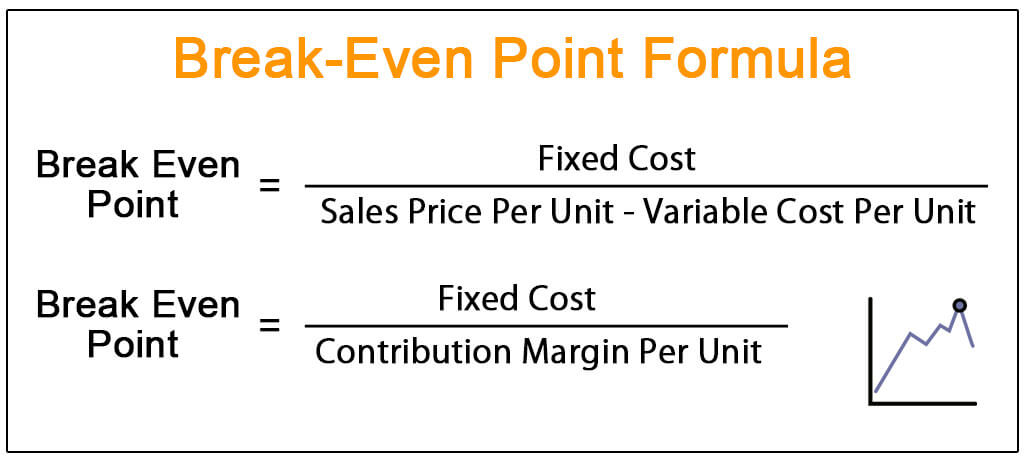Data processing in the USA: By clicking on "I accept", you also consent, in accordance with article 49 paragraph 1 sentence 1 lit. GDPR, to your data being processed in the USA (by Google LLC, Facebook Inc., LinkedIn Inc., Stripe, Paypal).

expert

Francesco

100% Recommendation Rate

3,414 Meetings

USD 449 / Coaching

3

# How to calculare the break even point for quantity and for sales?

Hello everyone,

I struggle with cases where I have to calculate the break-even point and I was wondering what approach do you suggest both for quantity and for sales so that I can understand it once and for all :-)

Thank you!

Hello everyone,

I struggle with cases where I have to calculate the break-even point and I was wondering what approach do you suggest both for quantity and for sales so that I can understand it once and for all :-)

Thank you!

• Date ascending
• Date descending

Hi there,

To find the quantity to breakeven, you can just rearrange the breakeven formula, which is the following:

R-VC-FC=0

⇔ p*q-c*q-FC=0

Where

• R= Revenues
• VC= Variable Costs
• FC = Fixed Costs
• p = Price
• q = Quantity
• c = Variable Cost per Unit

To calculate the quantity, you can rearrange as follows:

p*q-c*q-FC=0

⇔ q=FC/(p-c)

Hope this helps,

Francesco

Hi there,

To find the quantity to breakeven, you can just rearrange the breakeven formula, which is the following:

R-VC-FC=0

⇔ p*q-c*q-FC=0

Where

• R= Revenues
• VC= Variable Costs
• FC = Fixed Costs
• p = Price
• q = Quantity
• c = Variable Cost per Unit

To calculate the quantity, you can rearrange as follows:

p*q-c*q-FC=0

⇔ q=FC/(p-c)

Hope this helps,

Francesco

Hi there,

Breakeven is tough as there are so many scenarios! Just remember, all breakeven is, is solving for x, where x is some number to figure out how much of something we need.

The formula you create is specific to the case. So, we may say: We make \$20 profit per shoe, and we spent \$1,000 to create the shoe stand. How many shoes do we need to breakeven?

In this case, the formula is 1,000 = \$20 x ====> x = 50 shoes

Understood? Feel free to message if you'd like to go through multiple examples/scenarios!Hi there,

Breakeven is tough as there are so many scenarios! Just remember, all breakeven is, is solving for x, where x is some number to figure out how much of something we need.

The formula you create is specific to the case. So, we may say: We make \$20 profit per shoe, and we spent \$1,000 to create the shoe stand. How many shoes do we need to breakeven?

In this case, the formula is 1,000 = \$20 x ====> x = 50 shoes

Understood? Feel free to message if you'd like to go through multiple examples/scenarios!Hello!

There are multiple ways, but hte most common one is dividing all costs into the contribution margin.

For example, imagine you borrow 100 for a coffee machine, and you sell coffees for 1 USD, or which you have costs of 90 cents.

Contribution margin is 10 cents, and break even would be 100/ 0.1

Hope it helps!

Cheers,

Clara

Hello!

There are multiple ways, but hte most common one is dividing all costs into the contribution margin.

For example, imagine you borrow 100 for a coffee machine, and you sell coffees for 1 USD, or which you have costs of 90 cents.

Contribution margin is 10 cents, and break even would be 100/ 0.1

Hope it helps!

Cheers,

Clara

## Related case(s)

### Chinese Chess - Airline Business During COVID-19

Solved 4.0k times
Chinese Chess - Airline Business During COVID-19 Sky China, a government-backed Chinese airline, has recently seen profits plummet due to COVID-19. Profits are down 80% in the months of February and March, but are showing early signs of a rebound in April.  They've brought you in to first investigate what can be done immediatedly to prevent hemorrhaging cash and surive in the short-term. They are also looking to see how the current situation can be viewed as an opportunity, and what can be done to prepare for the future.
| Rating: (4.3 / 5.0)

Sky China, a government-backed Chinese airline, has recently seen profits plummet due to COVID-19. Profits are down 80% in the months of February and March, but are showing early signs of a rebound in April. They've brought you in to first investigate what can be done immediatedly to prevent hemor ... Open whole case